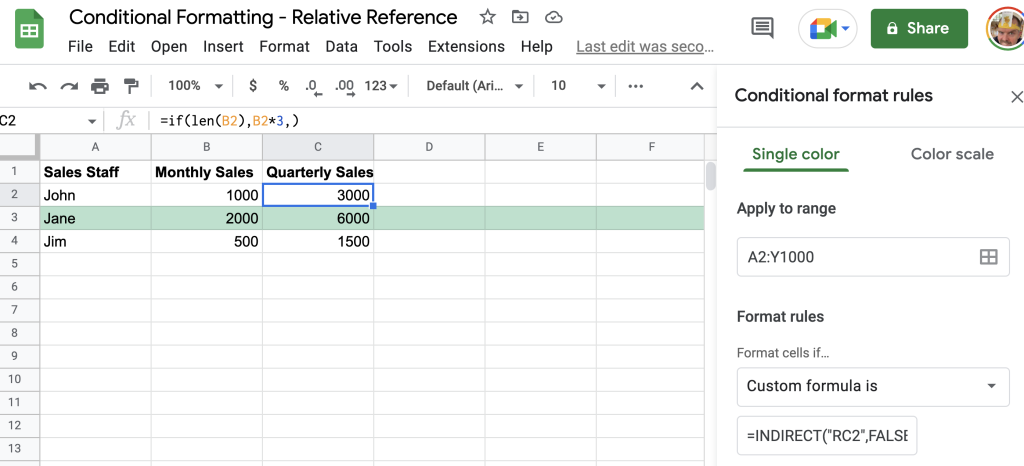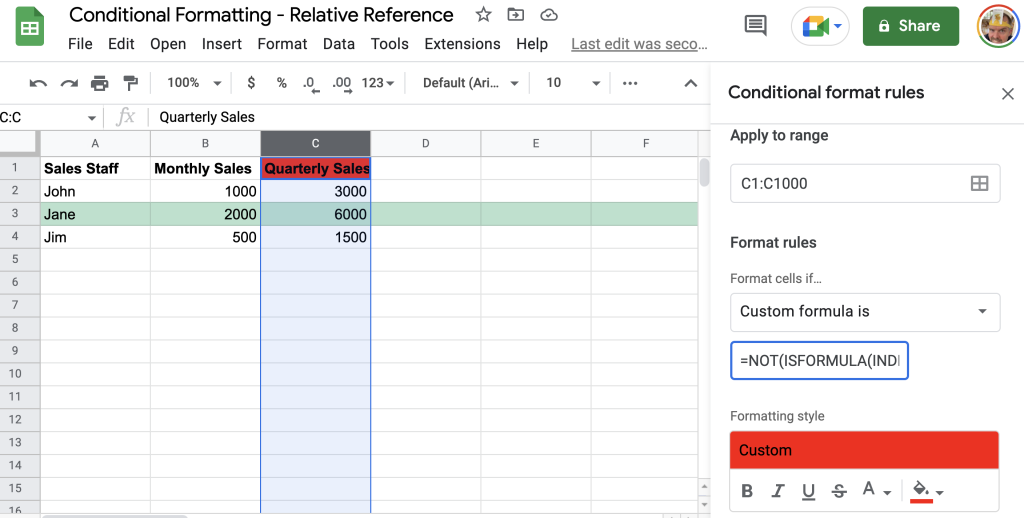# Conditional Formatting With Custom Formula Using Relative References

How can you apply conditional formatting using a custom formula that contains a relative reference to an adjacent row or column in Google Sheets?

Use Python Xlsxwriter To Create Exc...
Use Python Xlsxwriter To Create Excel Conditional Formatting(Part 4)

If you want to highlight a cell in Google Sheets using conditional formatting based on the condition of a nearby cell you can easily do so by using the Custom Formula feature along with the `INDIRECT()` formula that contains a relative reference.

The `INDIRECT(cell_reference, is_A1_notation)` formula in Google Sheets contains two parameters with the first parameter being a string representing the specific cell reference either as a named range (i.e. `salesTax`), or an absolute reference known by the common A1 notation with column letter followed by the row number (i.e. `A1`). The second parameter to the `INDIRECT` formula `is_A1_notation` is by default set to `TRUE`, therefore, when using a relative reference this parameter needs to be set to `FALSE`.

Here are some examples demonstrating each type of `INDIRECT()` formula call in a spreadsheet (cell `B1` is a named range cell labelled as `salesTax`):

As you can see from the above spreadsheet snippet the `INDIRECT()` formula is used in three distinct ways with the first showing how to use a named range `salesTax` which references cell `B1`, and the second showing an absolute reference using the A1 notation of `"B2"`, and the third using relative referencing.

## What Is A Relative Reference?

A relative reference is the opposite of an absolute reference. An absolute reference is a reference specifically pointing to a range that is either named (i.e. `salesTax`) or written in `A1` notation. In contrast a relative reference cell is a reference to a range that is a specific number of rows or columns away from the active cell.

As demonstrated above in the spreadsheet snippet the last `INDIRECT()` formula contained a reference using the letters `R` and `C` along with a number alongside each letter. Instead of knowing the name of the cell or the exact A1 notation you can obtain the value of the cell using a relative reference to the cell needed.

The instructions needed to get to the cell require you to know the distance from the active cell to the cell you want to reference. The distance must be measured in rows (up, as a positive number, or down, as a negative number) and columns (right, as a positive number, or left, as a negative number).

Therefore compared to the absolute cell references the syntax used with a relative reference must contain an annotation in row distance and column distance.

The way this is designated in Google Sheets is by using the `R` label for row distance and the `C` label for column distance with adjacent to each label a number in square brackets of the relative distance from the active cell.

For example, the relative reference of `RC` (which has nothing to do with R2D2’s cousin) from the active cell `A1` may indicate the cell to increase the row by 2 values and to increase the column by 2 values.

If you think this would reference `C3` you would actually be wrong. Why?

One major detail about the relative reference syntax is that the active cell has the relative reference `RC`. This could also be written as `R1C1` or even `RC`.

Therefore, when using the relative reference `RC` in effect you are only increasing the row by one (not two!) and the column reference by one (not two!). The relative reference will point to cell `B2`, not `C3`.

If you wanted to reference a row above the active cell or to the left of the active cell then you can use a negative number in square brackets to obtain the value of cells above (where you need to reduce the row value) or to the left of the active cell (where you need to reduce the column value of the cell).

What happens when you use the relative reference `R[-1]C[-1]` in cell `A1`?

### #REF! Error When Using Relative Reference

If you get a `#REF!` error when using a relative reference it could be due to two main factors:

You’re referencing a cell that cannot be found on the sheet. For example, if you’re in cell A1 and are referencing a row above the active cell, or referencing a column to the left of the active cell then because you are in the top and right-most cell there are no cells above and to the left.

Hence the `#REF!` error.

Another common reason for the `#REF!` error could be due to a circular reference. This could be the relative reference pointing to a cell which uses the active cell to help calculate its value, or you have entered into the relative reference any of the following values which indicate the active cell:

• `R1C1`
• `R[-1]C[-1]`
• `RC1`
• `R1C`
• `RC`
• `RC`

All of the above relative references mean the same active cell.

## Relative Cell Reference With Conditional Formatting

With the basics understood of what a relative reference looks like and how it behaves in Google Sheets, you can now use this syntax in your conditional formatting.

Knowing how a relative reference works in Google Sheets can help with a conditional formatting range where you might want to highlight an entire row or column based on a cell in the row triggering a condition.

For example, if you wanted to highlight an entire row based on whether a value in the row is greater or less than some other value you can use the relative reference with the INDIRECT formula.

First, select the entire sheet and then click on the Format main menu then click on Conditional Formatting option.

From here create a new conditional format and select Custom formula is for the condition.

In the custom formula field enter the formula:

`=INDIRECT("RC2", FALSE) > 1000`

This formula means that if the value in the second column across (to the right) is greater than the value of `1000` then perform the formatting.

This would look something like this:

Notice for the highlighting to work the range must be over the whole sheet, in my case above `A1:Y1000`. As the first header row is meeting the condition I would change the Apply to range cell to `A2:Y1000` to have it ignore the first row.

However, as you can see the relative reference works in highlighting the entire row where the condition is met (i.e. where the monthly sales column is greater than 1000).

## Highlight If Formula Does Not Exist In Cell

Another very popular conditional formatting technique I use is to check if a formula exists within a cell. This formatting can be very useful when you have important formulas and users tend to insert rows of data without knowing they need to copy the formulas in adjacent rows above.

With the same example of monthly sales staff if I added a column labelled `Quarterly Sales` that contained a simple formula of checking if the monthly sales value was present and if so to multiply that by 3, the formula would look something like this:

``=IF(LEN(B2),B2*3,)``

And I’ll copy this formula all the way to the end of the column. The sheet now looking like this:New column added with formula and conditional formatting not applying on top row A2:Y1000

To make sure a user of the spreadsheet doesn’t come in and insert a row of data and forget to apply the formula you can use the `ISFORMULA()` formula on the active cell relative reference.

To add this type of conditional formatting to your spreadsheet simply highlight the range where a formula should exist and in the Custom formula is field enter the following formula:

``=NOT(ISFORMULA(INDIRECT("RC", FALSE)))``

This should look like the following in your conditional formatting area on your spreadsheet:Highlighting a range red if it does not contain a formula

As you can see from the above screenshot the top cell is highlighted in red as it does not contain a formula, whereas the rest of the column is not as they all contain the quarterly sales projection formula.

Knowing that the header row will not contain a formula I can change the range from `C1:C1000` to `C2:C1000` to remove the conditional format applying to the header row.

## Summary

Conditional formatting can be a great way of being able to highlight and show the reader conditions being met, or something not being quite right. Knowing how to use relative referencing can help highlight an individual cell in a range, such as a cell not having a formula, or can be used to highlight an entire row.

Remember when using relative referencing in a conditional format to use the `INDIRECT("R[n]C[n]", FALSE)` formula where `R[n]C[n]` relates to the relative cell reference from the active cell.

If you’d like to see another conditional formatting example check this article where a custom formula based on a value in the first column is used to highlight the entire row.